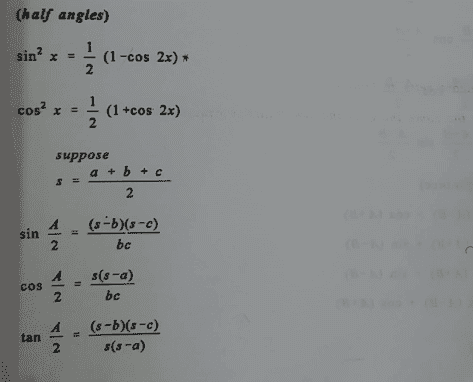# Proof of the trig identities for half-angles

• chwala

#### chwala

Gold Member
Homework Statement
see attached
Relevant Equations
TrigonometryI was just checking this out the sin##\frac {A}{2}## property, in doing so i picked a Right-Angled triangle, say ##ABC##, with ##AB=5cm##, ##BC=4cm## and ##CA= 3cm##. From this i have,
##s=6cm## now substituting this into the formula,
##sin\frac {A}{2}##= ##\frac {1×3}{5×3}##=##\frac {3}{15}##=##0.2##
giving us angle ##A=23.06^0## which does not look correct to me because,

angle ##A=53.13^0## ...using trigonometry directly...##sin{A}##= ##\frac {4}{5} =0.8##... i suspect a mix up in the indicated property...or i may have made a mistake.
i will amend my latex later using phone...

aaaaaaaaargh these guys are missing the square root sign! I had to check that from google.

Last edited:
The left hand sides should be squared or the right hand sides square-rooted
ie
$$\sin^2\left(\frac{A}{2}\right)=\frac{(s-b)(s-c)}{b c}$$
or
$$\sin\left(\frac{A}{2}\right)=\sqrt{\frac{(s-b)(s-c)}{b c}}$$

•chwala